Question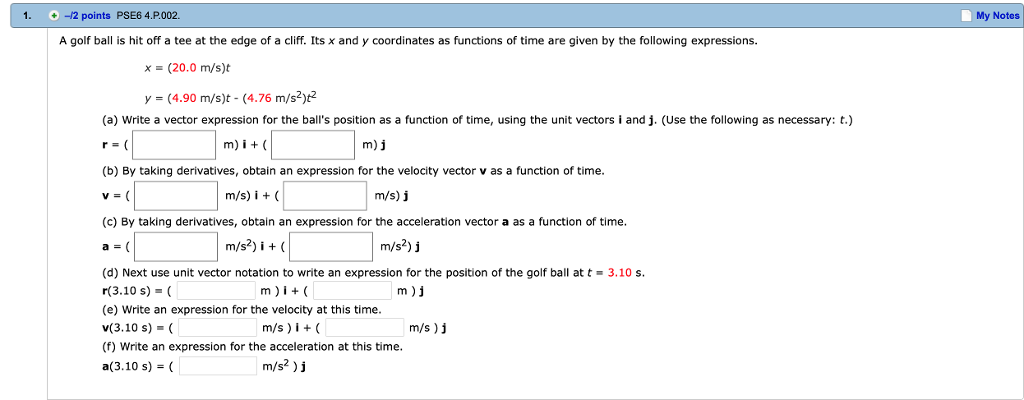If the number is a decimal please include 4 digits past the decimal point if available.

Given

x and y coordinate as:

x= (20.0 m/s) * t

y = ( 4.90 m/ s) * t - (4.76m/ s^2) *t^2

Part ( a )

position vector ( r ) is given as :

r = ​​​​​​x i + y j

r=(20* t m) i + {( 4.9* t - 4.76 * t^2 )m} j ......... eqn 1)

here m = meter

part (b)

v= deriveitive of position vector (r) w.r.t

v= d r/dt

v= 20 m/s i + {(4.90 -4.76 *2* t )m/s } j

v= 20m/s​​​​​​ i​​​​​​ + {(4.90-9.52*t) m/s} j ............... eqn 2)

part ( C)

a = dv /dt

a = 0 m/s^2 i + {(0 - 9.52)m/s^2} j

a =( -9.52 m/ s^2) j ...........eqn 3)

Part (d)

position vector of golf at t= 3.10m/s

r=( 20* 3.10) i + (4.90 * 3.10- 4.76 * 3.10^2) j

r=( 62 m) i +{( 15.19- 45.7436)m} j

r = (62m) i - {(30. 5536)m} j

part (e)

velocity vector at t= 3.10 s

v = 20 i + ( 4.90 - 9.52* 3.10) j ...... ( from eqn 2 )

v= (20m/s) i - {(24.612 ) m/ s } j

part (f)

a ( at t= 3.10 s)   from eqn 3 we have

a=( - 9.52 m/s^2) j ( it is independent of time)

#### Earn Coins

Coins can be redeemed for fabulous gifts.

Similar Homework Help Questions
• ### A golf ball is hit off a tee at the edge of a cliff. Its x...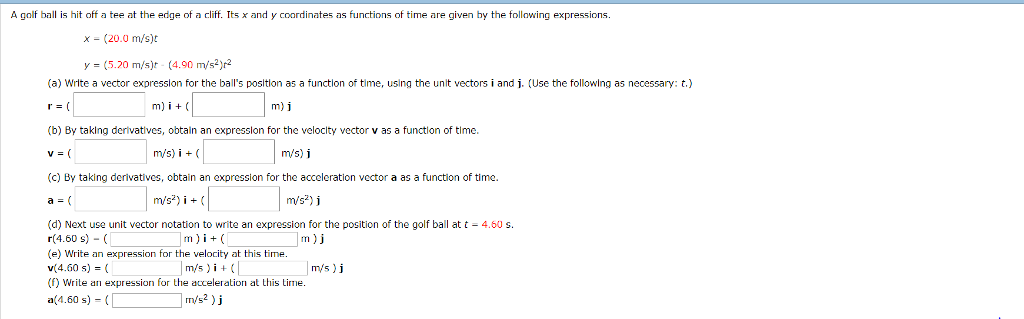A golf ball is hit off a tee at the edge of a cliff. Its x and y coordinates as functions of time are given by the following expressions. x- (20.0 m/s)t y (5.20 m/s)r-(4.90 m/s2)r2 (a) Write a vector expression for the ball's position as a function of time, using the unit vectors i and j. (Use the following as necessary: t.) (b) By taking derivatlves, obtain an expression for the velocity vector v as a function of time...

• ### A golf ball is hit off a tee at the edge of a cliff. Its x...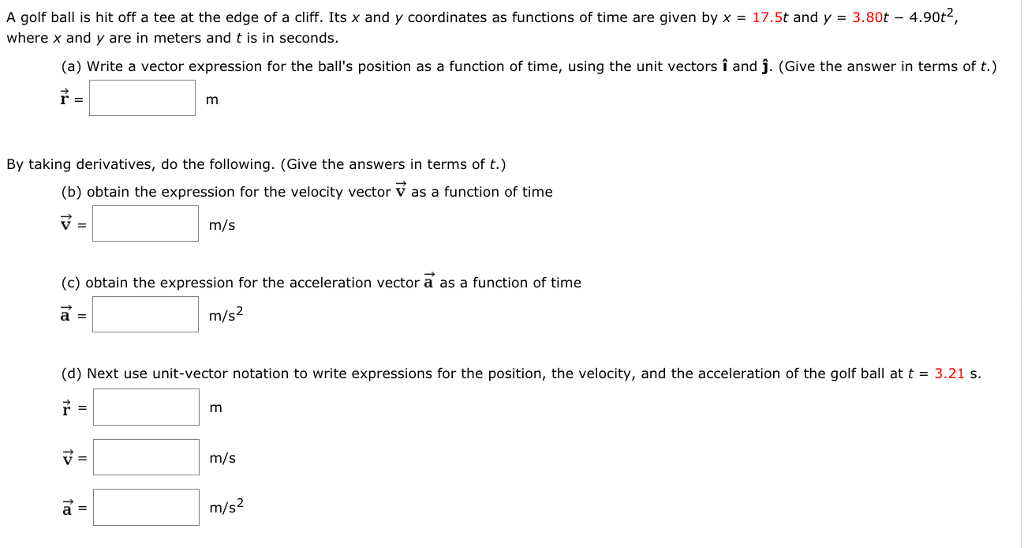A golf ball is hit off a tee at the edge of a cliff. Its x and y coordinates as functions of time are given by x = 17.5t and y = 3.80t-4.90t. where x and y are in meters and t is in seconds (a) Write a vector expression for the ball's position as a function of time, using the unit vectors i and j. (Give the answer in terms of t.) By taking derivatives, do the following. (Give...

• ### A golf ball is hit off a tee at the edge of a cliff. Its x...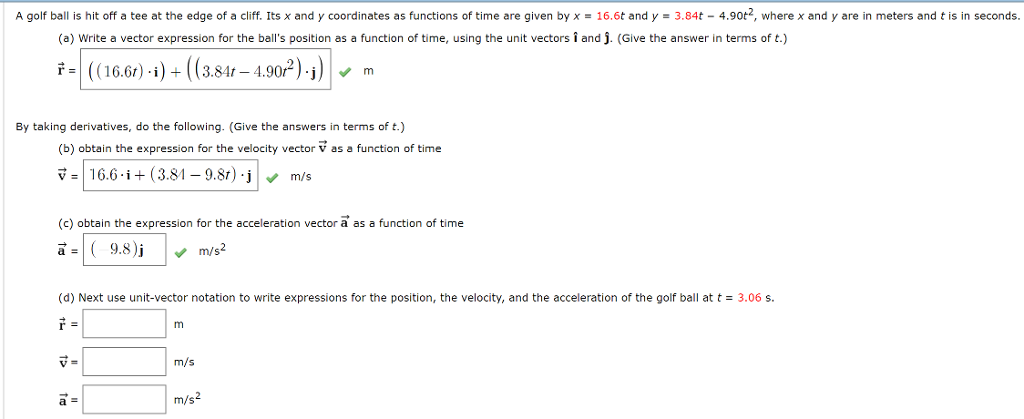A golf ball is hit off a tee at the edge of a cliff. Its x and y coordinates as functions of time are given by x = 16.6t and y = 3.84t − 4.90t2, where x and y are in meters and t is in seconds. (d) Next use unit-vector notation to write expressions for the position, the velocity, and the acceleration of the golf ball at t = 3.06 s. I need help with part d. A golf...

• ### 1A golf ball is hit off a tee at the edge of a cliff. Its x and y coordinates as functions of time are given byx = 18.1tandy = 3.64t −4.57t2,where x and y are in meters and t is in seconds.(a) Write a vector expression for the ball's position as a function of time, using the unit vectors and .(Give the answer in terms of t.) =By taking derivatives, do the following. (Give the answers in terms of t.)...

• ### Your last submission is used for your score -IG poinms SerPSES 4.P.005. My hotes Ask Your...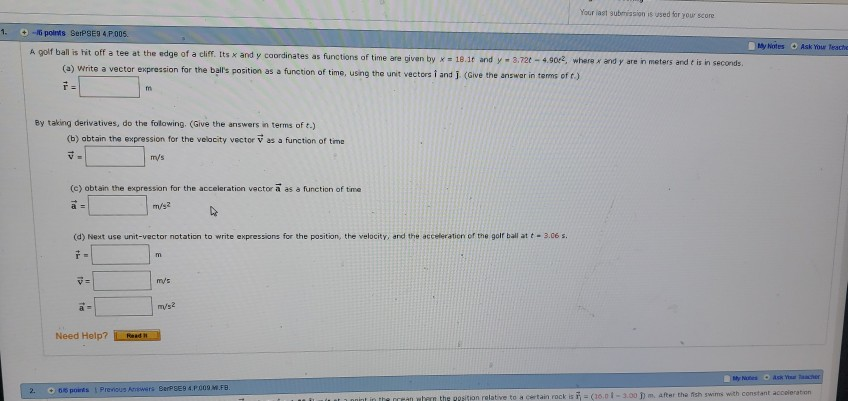Your last submission is used for your score -IG poinms SerPSES 4.P.005. My hotes Ask Your Teacho A golf ball is hit off a tee at the edge of a cliff. Its x and y coordinates as functions of time are given by x - 18.lt and y 3,72t-4.90, where x and y are in meters and t is in seconds (a) Write a vector expression for the ball's position as a function of time, using the unit vectors i...

• ### If the number is a decimal please include 4 digits past the decimal point if available....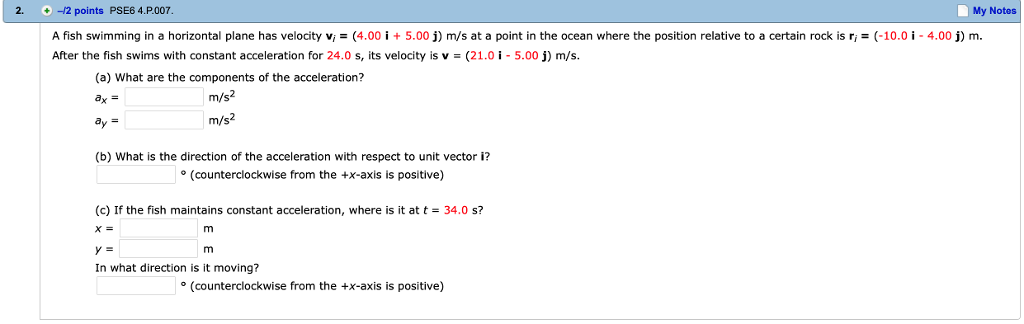If the number is a decimal please include 4 digits past the decimal point if available. 2. -12 points PSE6 4.P.007 My Notes A fish swimming in a horizontal plane has velocity vi = (4.00 İ + 5.00 j) m/s at a point in the ocean where the position relative to a certain rock is ri = (-10.01-4.00 j) m. After the fish swims with constant acceleration for 24.0 s, its velocity is v-(21.0-5.00) m/s. (a) What are the components...

• ### A polo ball is hit with a mallet off the edge of a cliff. Its x-...

A polo ball is hit with a mallet off the edge of a cliff. Its x- and y-coordinates as functions of time are given by x = 18.2t and y = 4.04t − 4.90t2, where x and y are in meters and t is in seconds. (Do not include units in your answer.) (a) Write a vector expression for the ball's position as a function of time (in m), using the unit vectors î and ĵ. (Give the answer in...

• ### If the number is a decimal please include 4 digits past the decimal point if available....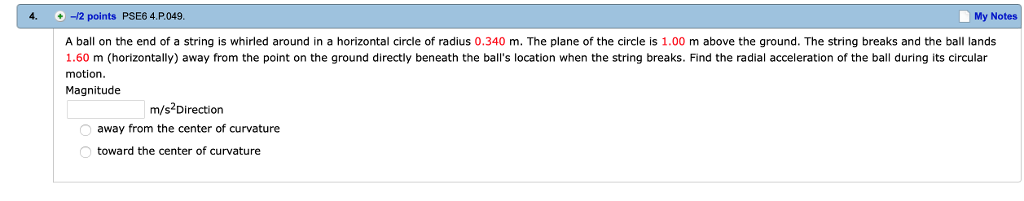If the number is a decimal please include 4 digits past the decimal point if available. 4. 2 points PSE6 4.P049 My Notes A ball on the end of a string is whirled around in a horizontal circle of radius 0.340 m. The plane of the circle is 1.00 m above the ground. The string breaks and the ball lands 1.60 m (horizontally) away from the point on the ground directly beneath the ball's location when the string breaks. Find...

• ### If the number is a decimal please include 4 digits past the decimal point if available....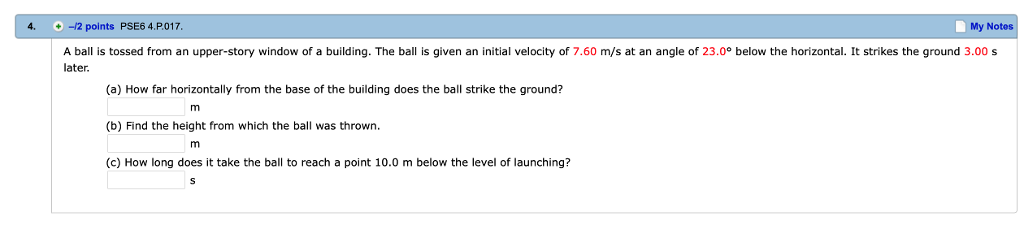If the number is a decimal please include 4 digits past the decimal point if available. 4. -12 points PSE6 4.P.017 My Notes A ball is tossed from an upper-story window of a building. The ball is given an initial velocity of 7.60 m/s at an angle of 23.0° below the horizontal. It strikes the ground 3.00 s later (a) How far horizontally from the base of the building does the ball strike the ground? (b) Find the height from...

• ### If the number is a decimal please include 4 digits past the decimal point if available....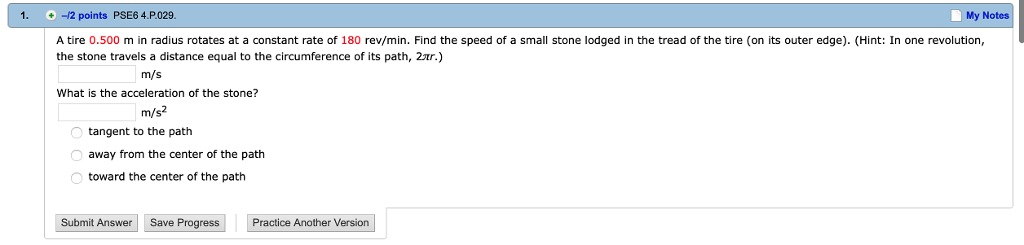If the number is a decimal please include 4 digits past the decimal point if available. 1. +-12 points PSE6 4.P.029. My Notes A tire 0.500 m in radius rotates at a constant rate of 180 rev/min. Find the speed of a small stone lodged in the tread of the tire (on its outer edge). (Hint: In one revolution, the stone travels a distance equal to the circumference of its path, 2ar.) m/s What is the acceleration of the stone?...